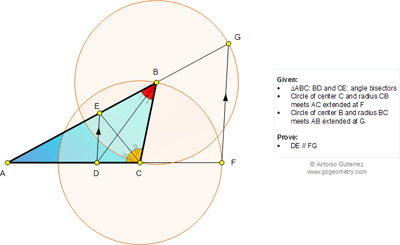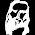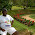## Sunday, May 4, 2014

### Geometry Problem 1011: Triangle, Angle Bisector, Vertex, Circle, Center, Radius, Parallel Lines

Level: Mathematics Education, High School, Honors Geometry, College.

Click the figure below to enlarge the figure of problem 1011.1.Let BC=a, AC=b, AB=c.

Then
AE = bc/(a+b)
EB = ac/(a+b)
DC = ab/(a+c)
AG = a+c
AF = a+b

Thus

Hence, DE//FG.

2.Below is geometry solution.
Note that ∠ (CBG)=1/2.( 180-∠A-∠C)=1/2. ∠B => DB//CG
Similarly EC//BF
∆AEC similar to ∆ABF => AE=(AB.AC)/ AF…… (1)
Divide ( 1) to (2) we get AE/AG=AD/AF => ED//FG

3.Problem 1011
Is <BCA=<CFB+<CBF or 2.<BCE=2.<BFC or <BCE-<BFC so CE//BF. Then AC/AE=AF/AB (1).
But <CBA=<BCG+<BGC or 2.<DBA=2.<CGB so DB//GC.Then AG/AC=AB/AD (2). With multiplication the (1) and (2) we have (AC/AE).(AG/AC)=(AF/AB).(AB/AD) or AG/AE=AF/AD. So DE//FG.
APOSTOLIS MANOLOUDIS 4 HIGH SCHOOL KORYDALLOS PIRAEUS GREECE

4.Let BC = CF = BG = a, AE =r, EB = s, AP = p and DC = q.

(r+s)/a = p/q ….(1) and
(p+q)/a = r/s ….(2)

From (1); (r+s+a)/a = (p+q)/q and
from (2); (p+q+a)/a = (r+s)/s and upon dividing

(r+s+a)/(p+q+a) = (p+q)/q X s/(r+s) = {(p+q)s}/{q(r+s)} = {ar}/{ap} =r/p

So AG/AF = AE/AD and hence DE // GF

Sumith Peiris
Moratuwa
Sri Lanka